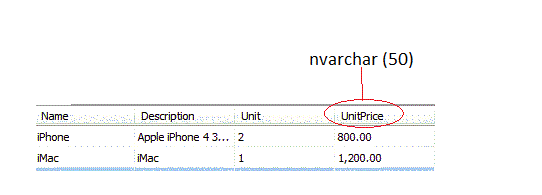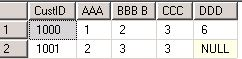# Convert nvarchar to float or number in sql

I recently came across the following usefull SQL query, Maybe you’ll find it useful.
In SQL, convert nvarchar (string) to number can be achieve by using cast and convert SQL function.
For example, I have Following tableYou may notice that UnitPrice has nvarchar datatype.
Here is my Query to convert UnitPrice datatype to float

```SELECT [Name]
,[Description]
,[Unit]
,convert(float,[UnitPrice]) [UnitPrice]
,[CreateDate]
FROM [Product]
```

Result:
Msg 8114, Level 16, State 5, Line 1
Error converting data type nvarchar to float.

Reason: Have a close look at the table, iMac UnitPrice is 1,200.00. UnitPrice 1,200.00 have coma which stop sql to convert into float.
Final Query:

```SELECT [Name]
,[Description]
,[Unit]
,convert(float,replace([UnitPrice],',','') )[UnitPrice]
,[CreateDate]
FROM [Product]
```

Thanks

# How to Convert rows into columns using Pivot in SQL Server(Query)

I recently came across the following usefull SQL query, Maybe you’ll find it useful.
In Sql, Pivoting can convert rows to columns. We will see these features in details in this post.
For example, we are considering a table named Ashish.want to perform a sql query to return results like this:# IF THEN in SQL SELECT Statement

I recently came across the CASE WHEN statement work Similar to IF statement into SQL SELECT , Maybe you’ll find it useful.Create table called Student using SQL Query:

```CREATE TABLE [dbo].[Student](
[StudentID] [int] NULL,
[Marks] [float] NULL
)
```

Insert some data into student:

```INSERT INTO [Student] ([StudentID],[Marks]) VALUES (1,30)
INSERT INTO [Student] ([StudentID],[Marks]) VALUES (1,65)
INSERT INTO [Student] ([StudentID],[Marks]) VALUES (1,90)
INSERT INTO [Student] ([StudentID],[Marks]) VALUES (1,55)
INSERT INTO [Student] ([StudentID],[Marks]) VALUES (1,70)
```

We can use CASE WHEN statement instead of IF. Here is Query:

```SELECT [StudentID]
,[Marks]
,case When [Marks] <65 and &#91;Marks&#93; >49
then 'Pass'
When [Marks] <75 and &#91;Marks&#93; >64
then 'Credit'
When [Marks] <85 and &#91;Marks&#93; >74
then 'Distinction'
When [Marks] <101 and &#91;Marks&#93; >84
then 'High Distinction'
else 'Fail'
end As Result
FROM [Student]
```

Thanks

# Sql Query to check file exists

I recently came across the following usefull SQL query, Maybe you’ll find it useful.

```declare @file_path	nvarchar(500)
declare @file_exists	int

set @file_path = 'C:\Temp.txt'

exec master.dbo.xp_fileexist
@file_path,
@file_exists output
Print	'File '+isnull(@file_path,'NULL')+' '+
case when @file_exists = 1
then 'exists'
else 'does not exist'
end
```

This actually check existence of file C:\Temp.txt

```Result:
File C:\Temp.txt exists
```

You can also use above query to check file Temp.txt file is exist. If YES Delete table or what ever…
Here is modified Query.

```declare @file_path	nvarchar(500)
declare @file_exists	int

set @file_path = 'C:\temp.txt'

exec master.dbo.xp_fileexist
@file_path,
@file_exists output

IF @file_exists = 1
BEGIN
DELETE FROM [Ashish].[dbo].[Temp]
END
```

Thanks.

# Trim not removing spaces in SQL

When I Was importing text file to SQL server, sometime it include spaces with data as well. And That space it not remove by using Trim function.These spaces appear as white spaces to our eyes but this is not the truth. When I check it with ASCII(char) it shows char 10- Line Feed(LF), 13 – Carriage Return(CR), 160 –nbsp. So the solution is to replace these characters with white spaces (CHAR(32)) and then apply the TRIM function to it. Below is the screipt for it:

```SELECT LTRIM(RTRIM(REPLACE(REPLACE(REPLACE([ColumnName], CHAR(10), CHAR(32)),
CHAR(13), CHAR(32)), CHAR(160),
CHAR(32)))) AS [ColumnName]
FROM [TableName]
```

Update:: include tab – CHAR(9)

```SELECT LTRIM(RTRIM(REPLACE(REPLACE(REPLACE(REPLACE([ColumnName], CHAR(10), CHAR(32))
,CHAR(13), CHAR(32))
,CHAR(160), CHAR(32))
,CHAR(9),CHAR(32))))
FROM [TableName]
```

Thanks